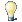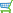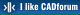#CAD tip # 10721:%  platform  category## How to draw a catenary or other parametric curve in Inventor?The catenary curve (also called a chainette, alysoid, funicular, see wiki) - the curve of hanging chain or cable or other fibre in a homogenous gravitational field - is used in many design tasks. To create a sketch shapes as a catenary in Inventor you can zse the function "Equation curve" and specify the curve parametrically.

On the above linked wiki page you can find the generalized equation of a planar catenary curve:

y = a * cosh (x / a)

where a is the parameter of uniform scaling of the curve.

Start the function Equation curve (Sketch > Create > Line > Equation curve):and enter the equation directly to the equation fieleds - choose the equation option "Explicit" and "Cartesian". Just make sure the correct units are combined - i.e. convert the length value of "x" to angle (mm->rad). Using the value of a = 1.8 and the range x -2 to 2:

y = 1.8 * cosh (x / 1 mm * 1 rad / 1.8)For possible subsequent editing of the curve, you the function "Edit equation curve" from the context menu in the model browser tree.

 Inventor*  CAD 31.1.2016    10754× Prices - CAD eShop:InventorProduct Design Collectionapplies to: Inventor ·

Have we helped you? If you want to support the CAD Forum web service, consider buying one of our CAD applications, or our custom software development offerings, or donating via PayPal (see above). You may also add a link to your web - like this "fan" link: